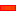﻿ MechDesigner Reference & User Interface > Dialog-Boxes > Dialog: Blend-Curve

# Dialog: Blend-Curve

Navigation:  MechDesigner Reference & User Interface > Dialog-Boxes >

# Dialog: Blend-Curve

## Blend-Curve

 A Blend-Curve sketch-element has a start-Point and an end-Point. You can use the Blend-Curve dialog-box to edit these geometric-properties AT its start-Point and AT its end-Point: •Angle •Curvature •Curvature Rate   Typically, you will merge the start-Point and/or end-Point of the Blend-Curve with another sketch-elements. The default geometric-properties ensure geometric continuity of the Blend-Curve with the adjacent sketch-elements. Case 0 : The Blend-Curve is not merged with another sketch-element. You can edit these geometric-properties at its start-Point and end-Point: •Angle (default = 0) •Curvature (default = 0) •Curvature Rate (default = 0) Default values Case 1 : You merge the start-Point and/or end-Point of the Blend-Curve with a Line. •Angle is equal to the angle of the Line •Curvature is equal to zero •Curvature Rate  is equal to zero Case 2 : Merge the start-Point and/or end-Point of the Blend-Curve with an Arc. •Angle is equal to the tangent of the Arc •Curvature is equal to 1 / Radius of the Arc (m) •Curvature Rate - is equal to zero Case 3 : Merge the start-Point and/or end-Point of the Blend-Curve with a Blend-Curve. Edit the end-Point of a Blend-Curve that you merge with the start-Point of an adjacent, or following, Blend-Curve. In the Blend-Curve dialog-box, edit the: •Angle : Disable the end-Point Angle check-box: edit the Angle - the angle of the adjacent Blend-Curve with equal the angle you enter. •Curvature : Disable the end-Point Curvature check-box: edit the Curvature - the curvature of the adjacent Blend-Curve with equal the curvature you enter. •Curvature-Rate : Disable the end-Point Curvature-Rate check-box: edit the Curvature-Rate - the curvature-rate of the adjacent Blend-Curve with equal the curvature-rate you enter. You can edit the geometric-properties •Exactly : with the Blend-Curve dialog-box •Approximately : with the Blend-Curve drag-handles.

### Edit ExactlyHow to open the Blend-Point dialog-box

The Blend-Curve dialog-box is now open.Blend-Curve dialog-box

### Edit ApproximatelyTo show / hide the Blend-Curve drag-handlesBlend-Curve Drag-Handles

Note on Curvature and Curvature-RateCurvature and Curvature-Rate